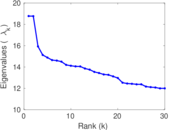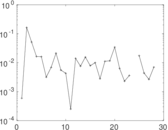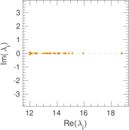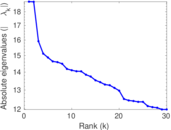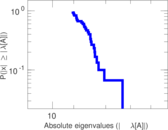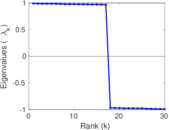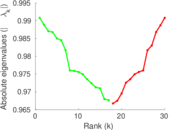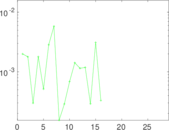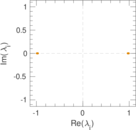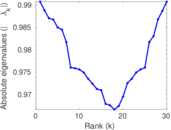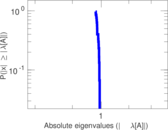# DBpedia

This is the complete DBpedia network. Nodes are entities included in DBpedia and each edge represents one triple. The data is extracted from version 3.6 of DBpedia.

 Code `DB` Internal name `dbpedia-all` Name DBpedia Data source http://wiki.dbpedia.org/Downloads AvailabilityDataset is available for download Consistency checkDataset passed all tests Category Miscellaneous network Dataset timestamp 2001 ⋯ 2017 Node meaning Concept Edge meaning Relationship Network format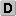Unipartite, directed Edge typeUnweighted, multiple edges ReciprocalContains reciprocal edges Directed cyclesContains directed cycles LoopsDoes not contain loops

## Statistics

 Size n = 3,966,924 Volume m = 13,820,853 Unique edge count m̿ = 12,860,157 Loop count l = 0 Wedge count s = 174,275,329,593 Claw count z = 19,647,361,248,950,896 Cross count x = 2.121 93 × 1021 Triangle count t = 8,329,548 Square count q = 31,569,203,235 4-Tour count T4 = 949,680,166,216 Maximum degree dmax = 472,799 Maximum outdegree d+max = 1,006 Maximum indegree d−max = 472,799 Average degree d = 6.968 05 Fill p = 8.172 31 × 10−7 Average edge multiplicity m̃ = 1.074 70 Size of LCC N = 3,915,921 Size of LSCC Ns = 178,593 Relative size of LSCC Nrs = 0.045 020 5 Diameter δ = 67 50-Percentile effective diameter δ0.5 = 4.675 03 90-Percentile effective diameter δ0.9 = 6.270 44 Median distance δM = 5 Mean distance δm = 5.189 84 Gini coefficient G = 0.661 842 Balanced inequality ratio P = 0.253 558 Outdegree balanced inequality ratio P+ = 0.339 768 Indegree balanced inequality ratio P− = 0.157 443 Relative edge distribution entropy Her = 0.874 373 Power law exponent γ = 1.940 85 Tail power law exponent γt = 2.071 00 Degree assortativity ρ = −0.042 546 6 Degree assortativity p-value pρ = 0.000 00 In/outdegree correlation ρ± = −0.127 840 Clustering coefficient c = 0.000 143 386 Directed clustering coefficient c± = 0.150 192 Spectral norm α = 731.842 Operator 2-norm ν = 729.740 Cyclic eigenvalue π = 18.770 8 Spectral separation |λ1[A] / λ2[A]| = 1.003 51 Reciprocity y = 0.038 751 5 Non-bipartivity bA = 0.005 699 68 Normalized non-bipartivity bN = 0.000 326 204 Algebraic non-bipartivity χ = 0.000 674 948 Spectral bipartite frustration bK = 2.626 81 × 10−5 Controllability C = 2,700,982 Relative controllability Cr = 0.680 881

## Plots

### Degree distribution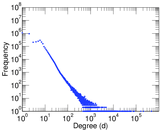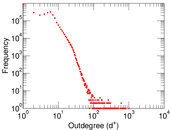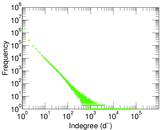### Cumulative degree distribution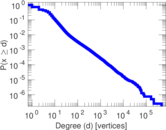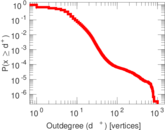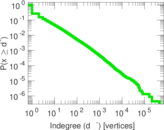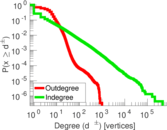### Lorenz curve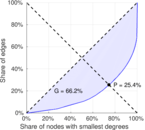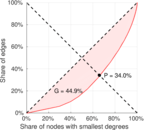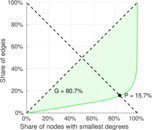### Spectral distribution of the adjacency matrix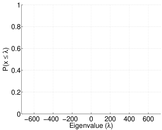### Spectral distribution of the normalized adjacency matrix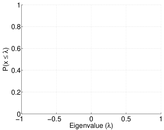### Spectral distribution of the Laplacian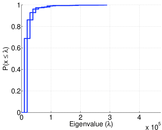### Spectral graph drawing based on the adjacency matrix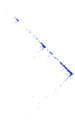### Spectral graph drawing based on the normalized adjacency matrix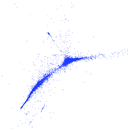### Degree assortativity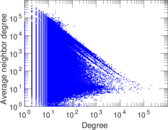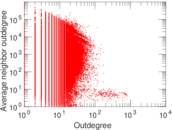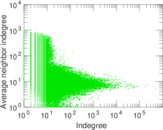### Hop distribution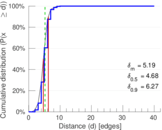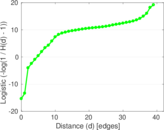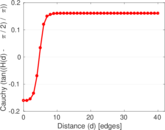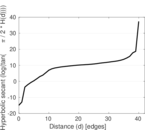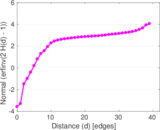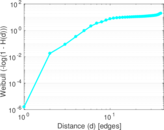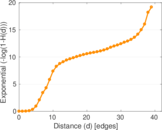### In/outdegree scatter plot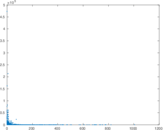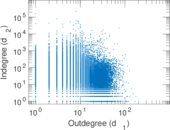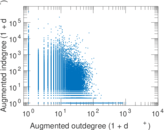### Edge weight/multiplicity distribution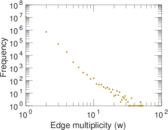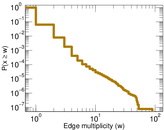### Clustering coefficient distribution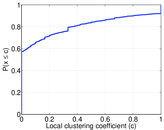### Matrix decompositions plots